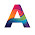9TH MAHARASHTRA: MATHS I 10TH TAMIL NADU: MATHS, SCIENCE, SSLC 10TH NEW TEXT BOOK PDF DOWNLOAD CBSE 10TH: MATHS 2019 BOARD PAPER SOLUTION: 2019 BOARD PAPER SOLUTION

WHAT ARE THE TYPES OF PRICE ELASTICITY OF DEMAND? Very important

Meaning: -Price elasticity of demand is generally known as elasticity of demand. Elasticity of demand show how much expansion and contraction of demand takes place due to fall or rise in price. Elasticity of demand is the proportionate change in quantity demanded of a commodity as a result of proportionate change in its price. In other words, Elasticity of demand is the ratio of percentage change in quantity demanded of a commodity to the percentage change in its price.

Symbolically,
Ed =   %change in quantity demanded
% change in price

The following are the different types of price elasticity of demand:

1.      Unitary Elastic Demand: - When a change in price of commodity brings about change in the demand of that commodity is exactly the same proportion, it is called as unitary elastic demand. For instance, a 10% rise in price of a commodity leads to a 10% fall in demand of that commodity.

Ed =    %change in quantity demanded    =  1
% change in price

2.      Relatively Elastic Demand: -When a change in price of a commodity brings about more than proportionate change in the demand of that commodity, it is called as relatively elastic demand. For instance, a 10% rise in price of a commodity leads to 20% fall in demand of that commodity.

Ed =    %change in quantity demanded    ≥ 1
% change in price

3.      Relatively Inelastic Demand: -When a change in price of a commodity brings about lessthan proportionate change in the demand of that commodity, it is called as relatively inelastic demand. For instance, a 10% rise in price of a commodity leads to 5% fall in demand of that commodity.

Ed =    %change in quantity demanded    ≤  1
% change in price

4.      Perfectly(Infinite) in elastic demand: -When a slight change in the price of a commodity brings about an infinite change in the demand of that commodity, it is called as Perfectly elastic demand. For instance, a 10% rise in price of a commodity may lead to infinite fall in demand of that commodity. The perfectly elastic demand curve is parallel to X-axis.

Ed =   %change in quantity demanded     =  ∞
% change in price

5.      Perfectly Inelastic demand: - When a change in price has noeffect on the quantity demanded of that commodity, it is called as perfectly inelastic demand. For instance, a 10% rise in price will have not effect on the quantity demanded. The quantity demanded remains the same irrespective of rise or fall in price of that commodity.

Ed =    %change in quantity demanded      = 0
% change in price

1.Is question me diagram नाही बनाया तो चलेगा?????

1.No Diagram is necessary.

2.Diagram is necessary or not?

2.I think diagrams are important if this answer comes for 8mrks.